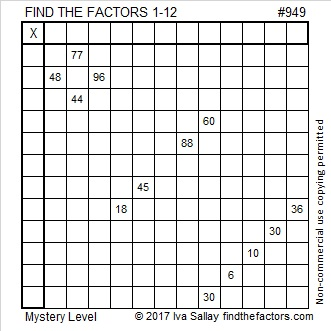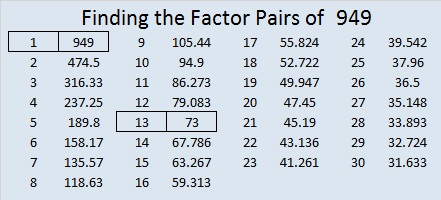# 949 Mystery Level

The difficulty level of a mystery level puzzle is a mystery. You won’t know how hard or how easy it is until you give it a try. You can solve it by using logic and your knowledge of the multiplication table. Can you figure it out?Print the puzzles or type the solution in this excel file: 12 factors 942-950

Now here is a little about the number 949:

948 and 949 are a Ruth-Aaron pair.

949 is not only a palindrome in base 10, but it is also
434 in BASE 15 because 4(15²) + 3(15¹) + 4(15⁰) = 949

25² + 18² = 949 and 30² + 7² = 949 so 949 is the hypotenuse of four Pythagorean triples:

301-900-949 calculated from 25² – 18², 2(25)(18), 25² + 18²
365-876-949 which is (5-12-13) times 73
420-851-949 calculated from 2(30)(7), 30² – 7², 30² + 7²
624-715-949 which is 13 times (48-55-73)

• 949 is a composite number.
• Prime factorization: 949 = 13 × 73
• The exponents in the prime factorization are 1 and 1. Adding one to each and multiplying we get (1 + 1)(1 + 1) = 2 × 2 = 4. Therefore 949 has exactly 4 factors.
• Factors of 949: 1, 13, 73, 949
• Factor pairs: 949 = 1 × 949 or 13 × 73
• 949 has no square factors that allow its square root to be simplified. √949 ≈ 30.80584This site uses Akismet to reduce spam. Learn how your comment data is processed.